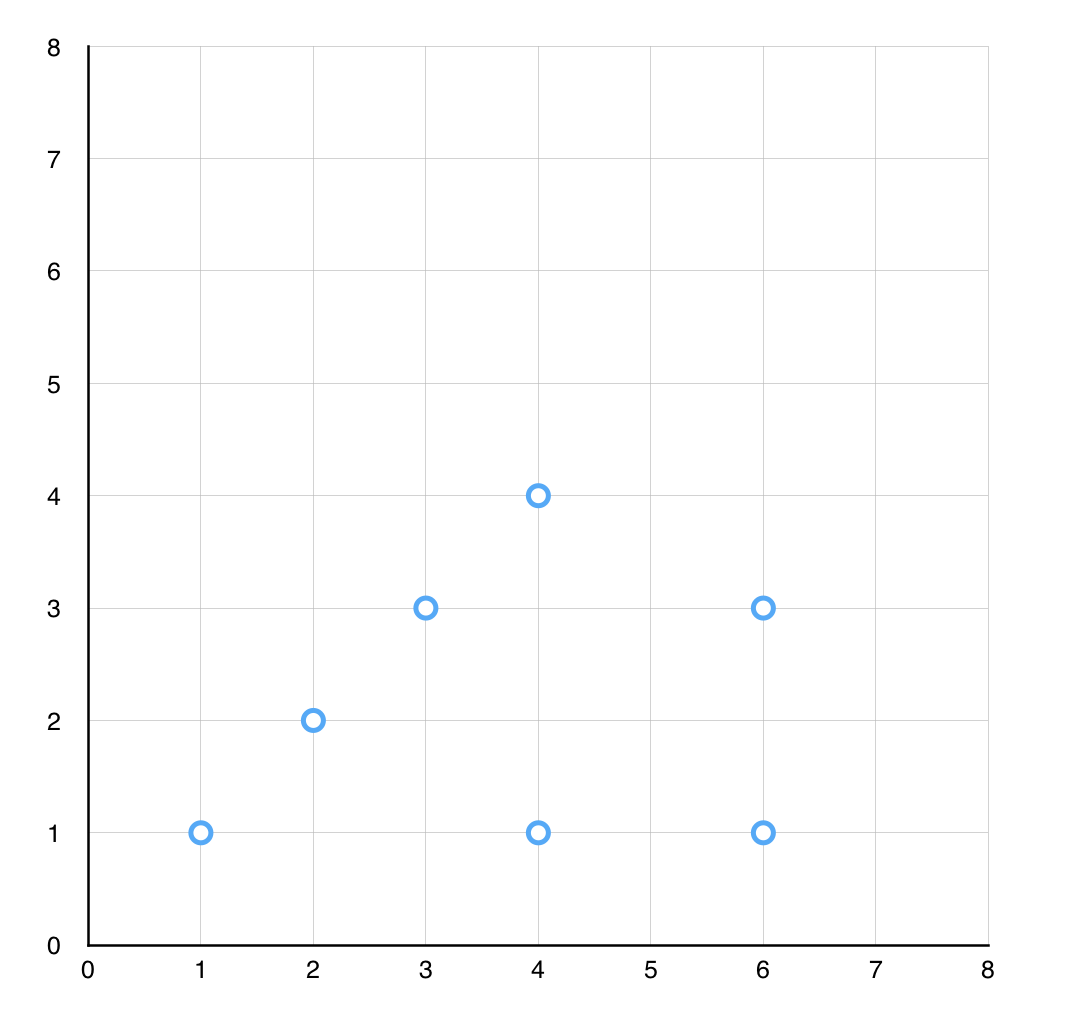Given n points on a 2D plane, find the maximum number of points that lie on the same straight line. For example, consider the following points.The maximum number of points over the same straight line is 4. Those points are (1,1), (2,2), (3,3), and (4,4).

Input. The first line consists of an integer, n, that indicates the number of points you will be given. Each of the following n lines consists of a pair of integers separated by one or more spaces corresponding to the x and y components of a point. Consider the following example.

Output.

The output consists of a single number, the maximum number of points that lie on the same straight line. For the example input, the maximum is 4.

# Solution

Here is a Ruby implementation.